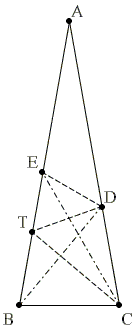# The 80-80-20 Triangle Problem, Solution #9Let ABC be an isosceles triangle (AB = AC) with ∠BAC = 20°. Point D is on side AC such that ∠CBD = 50°. Point E is on side AB such that ∠BCE = 60°. Find the measure of ∠CED.

Solution

This is Solution 8 from [Knop] and is due to Sergey Saprikin, a high school student at the time.

Let T be the intersection of the bisector of angle ACB with the side AB.Then ∠BTC = 60°, and, since BC = CD, ΔBCT = ΔDCT. Hence ∠CTD = 60°.

In ΔCDT, E is the intersection of the angle bisector at C and of the external angle bisector T. T is then one of the excenters of ΔCDT. It follows that, in that triangle, DE is the external bisector of the angle at D. ∠BDT = ∠DBT = 30°. Next, ∠CDT = 80°, ∠EDT = (180° - 80°)/2 = 50°, and, finally, ∠CED = 180° - 20° - 50° - 30° - 50° = 30°.

### Reference

1. C. Knop, Nine Solutions to One Problem, Kvant, 1993, no 6.### The 80-80-20 Triangle Problem